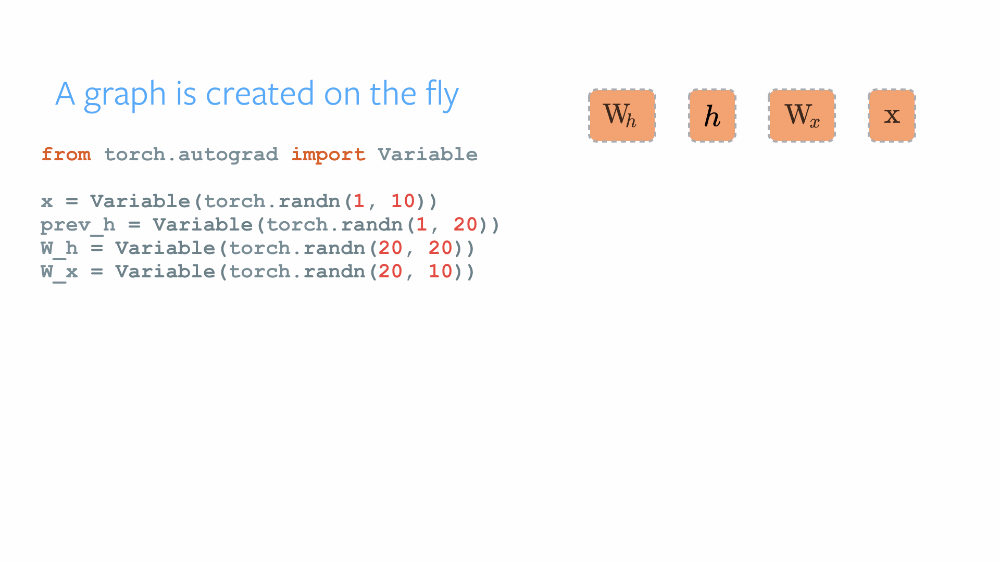pytorch学习笔记（十三）：backward过程的底层实现解析

pytorch源码注释，欢迎 pr，提 issue 和 star

• pytorch 是如何动态构建反向传导图的
• pytorch 的反向传导是怎么操作的

pytorch 是如何构建反向传导图的// Add 函数 计算方法的实现， 构建反向传导图的关键在 wrap_outputs
auto Add::apply(const variable_list& inputs) -> variable_list {
auto& input1 = inputs->data;
auto& input2 = inputs->data;
AutoGPU guard(input1->getDevice());

bool first_sparse = input1->isSparse();
auto output = first_sparse ? input2->newTensor() : input1->newTensor();
if (first_sparse) {
} else {
}

return wrap_outputs(inputs, as_tensor_list(std::move(output)), [&](FunctionFlags f) {
});
};

• 根据 forward 过程中的 inputs 来计算 backward 函数的 flag （is_volatile, is_executable, next_functions）
• 然后将 forward 的输出 的 grad_fn 设置成 创建好的 backward 函数。
• 这样，函数节点就构成了一张 反向传导图！（通过不停的 .next_functions.next_functions）

variable_list wrap_outputs(const variable_list& inputs, tensor_list&& outputs,
function_constructor ctr) {

// 使用 inputs variables 来计算 反向传导的 Function 的 flag (f.is_executable, f.is_volatile)和 next_functions
// 这里需要搞清楚的一点是：inputs 是前向传导的 inputs，从它可获得的信息有：当前 函数的 反向传导函数 是否 可执行，

auto flags = Function::flags(inputs);
variable_list result;

// 开始创建 返回的 Variable 了。
result.reserve(outputs.size());

if (flags.is_volatile) {  // 如果 is_volatile=true, 那么输出的 Variable 的 is_volatile=true
for (auto& output : outputs) {
if (output.defined()) { // 因为 可能返回 None 嘛，所以这里 check 一下
result.emplace_back(make_variable(output, false, true)); // requires_grad=false, is_volatile=true
} else {
result.emplace_back();
}
}
} else {  // 如果 volatile=false， 难道也不管 is_executable 了吗？
// ctr 是一个 lambda 函数， 它返回一个 std::shared_ptr<GradFn>
// 梯度 使用 Function::flags 计算出来的 flags 其实是给 Backward 用的。
auto grad_fn = ctr(std::move(flags));  // 用 flags(is_executable, is_volatile) 创建出来一个 Function。
for (auto& output : outputs) {
if (output.defined()) {
} else {
// forward 的输出 变量个数，就是 backward 的输入变量个数。
result.emplace_back();
}
}
}
return result;
}

pytorch 的反向传导计算过程

a = o+e
c = a+b
d = a+e
res = c+d

1. 创建了一个新的 结构体 FunctionTask， 里面有个 InputBuffer 属性，这个是用来累积来自不同路径的梯度的
2. 什么时候才累积完呢？ pytorch 对每个 grad_fun 节点都求了其依赖 , 比如 上例中的 grad_fn(a,o,e) 的依赖就是 2, 因为，a 被用了两次。 grad_fn(a,o,e) 没聚集一次梯度，其依赖就 -1, 当依赖为 0 的时候，就将其对应的 FunctionTask 放到 ready_queue 中等待 被执行。

backward 过程用到的一些 数据结构

struct FunctionTask {
InputBuffer inputs; // 累积 grad_fn 的输入

: base(base)
, fn(fn)
, inputs(std::move(inputs)) {}
};
struct ReadyQueue {
// queue 是 FunctionTask 的 一个 双端队列
// std::condition_variable 条件变量，同步的时候会用到
// 用 unique_lock (over mutex) 来进行操作
std::condition_variable not_empty;
std::mutex mutex;

};
struct GraphTask {
// 记录整个反向计算图的依赖情况 等等。
std::exception_ptr exception;
// Indicates if an error occurred while executing any task.  When this is
// true, it signals all threads to stop executing.
std::atomic_bool has_error;

bool keep_graph;
bool has_any_work;
// 用来 给 notify_all 加锁的
std::mutex mutex;
// if all tasks are done.
std::condition_variable not_done;
const Engine::pre_callback_map& pre_callbacks;
const Engine::post_callback_map& post_callbacks;

};
struct Engine{
}